## PERLIN

 Creates an image composed of Perlin type noise.

 USAGE: perlin widthxheight [-s startlevel] [-e endlevel] [-b base] [-a attenuation] [-n noise] [-r reseed] [-f filter] [-m mode] outfile USAGE: perlin [-h or -help] ....... widthxHeight ....... width and height of desired output image -s .... startlevel ......... starting octave level; integer>0; default=1 -e .... endlevel ........... ending octave level; integer>0; default is ............................ as high as practical -b .... base ............... octave base; integer>1; default=2 -a .... attenuation ........ attenuation factor; float>=0; default=0 ............................ indicates attenuate linearly; other values ............................ indicates use an exponential attenuation with ............................ the value as the base; attenuation=2 is the ............................ other nominal value -n .... noise .............. noise type; any valid IM noise type; ............................ default=random -r .... reseed ............. seed value for random number generator; integer>=0; ............................ default indicates to use a random seed value ............................ each iteration and each time the script is run -f .... filter ............. noise smoothing interpolation filter; ............................ any valid IM filter; default=lanczos -m .... mode ............... output image color mode; gray or color; ............................ default=gray PURPOSE: To create an image composed of Perlin type noise. DESCRIPTION: PERLIN creates an image composed of Perlin type noise. Perlin noise is an addition of random noise images at successively higher octaves in frequency and more attenuated amplitudes. ARGUMENTS: widthxheight ... WIDTHxHEIGHT is the desired sized of the output image. -s startlevel ... STARTLEVEL is the starting octave level. It defines the lowest frequency of the random pattern (added to zero frequency midgray) where the frequency is the base raised to the octave level. Values are integers>0. The default=1. -e endlevel ... ENDLEVEL is the ending octave level. It defines the highest frequency of the random pattern where the frequency is the base raised to the octave level. Values are integers>0. The default is to stop when the amplitude is smaller than the IM smallest number, quantumscale, for your IM Q level compilation. Practically, one might want to stop at octave level around 6 or 7 depending upon output size. If the endlevel is too high, then the resulting noise pattern may be too fine-grained. -b base ... BASE is the base number for the octave frequencies. Frequencies are defined as base raised to the power of the octave level. Values are integers>1. The default=2 (frequencies increment as octaves that are powers of 2). Typical values are small numbers. -a attenuation ... ATTENUATION is a value used to compute how the amplitude for each octave is attenuated (or amplified) as a function of increasing noise frequency. It is the inverse of what is typically called the "persistence" in some of the literature. Values are floats>0. For the default value of zero, the amplitude will be attenuated by successively larger integers incremented for each successive octave. When the attenuation value is larger than zero, the amplitude will be attenuated by the attenuation value raised to the same integer. Thus for values greater than 1, higher frequencies will be attenuation. But for values less than 1, higher frequencies will be amplified. Each frequency will carry the same weight, when the attenuation=1. A value of 2 is often used and produces a smoother result than the default of 0. -n noise ... NOISE is the type of noise to use. Any valid IM noise is permitted. The default=random. -f filter ... FILTER is the smoothing or interpolation filter used to generate the lower frequency patterns. Any valid IM filter is permitted. The default=lanczos. -r reseed ... RESEED is the seed value to use in the random number generator. This permits the resulting image to be repeated. Values are integers>=0; The default is to change the seed value randomly each time the script is run and for each octave. When a seed value is provided, it will be used for all octaves. REQUIREMENTS: IM 6.5.5.-1 or higher due to the use of -auto-level. CAVEAT: No guarantee that this script will work on all platforms, nor that trapping of inconsistent parameters is complete and foolproof. Use At Your Own Risk.

### EXAMPLES

 Variation In Seed Value Arguments: -r 100 Arguments: -r 200 Arguments: -r 300 Arguments: -r 400Variation In End Level Octave Arguments: -e 7 Arguments: -e 6 Arguments: -e 5 Arguments: -e 4 Arguments: -e 3Variation In Start Level Octave Arguments: -s 1 -e 7 Arguments: -s 2 -e 7 Arguments: -s 3 -e 7 Arguments: -s 4 -e 7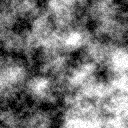Variation In Base Arguments: -b 2 Arguments: -b 3 Arguments: -b 4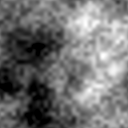Variation In Attenuation Value (Persistence) Arguments: -a 0 (default) Arguments: -a 1 Arguments: -a 1.5 Arguments: -a 2 Arguments: -a 3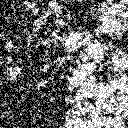Variation In Type Of Noise Arguments: -n random -a 0 (default) Arguments: -n gaussian -a 0 Arguments: -n poisson -a 0Arguments: -n random -a 2 (default) Arguments: -n gaussian -a 2 Arguments: -n poisson -a 2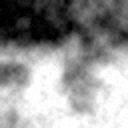Variation In Filter Arguments: -f lanczos (default) Arguments: -f triangle Arguments: -f cubic Arguments: -f gaussian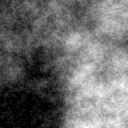Color Arguments: -a 0 -m color Arguments: -a 2 -m color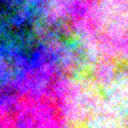What the script does is as follows: Computes a mid gray image for the zero frequency octave Computes a random small image of size base^startlevel, then enlarges it to the desired size, subtracts 0.5 and multiples by the attenuated amplitude, then adds that to the zero frequency image Repeats for each successive octave until the endlevel is reached This is equivalent to the following IM commands for mode=gray maxwh=`convert xc: -format "%[fx:max(\$width,\$height)]" info:` dim=`convert xc: -format "%[fx:\$base^(\$startlevel)]" info:` maxdim=`convert xc: -format "%[fx:\$base^ceil(log(\$maxwh)/log(\$base))]" info:` i=\$startlevel j=1 stop=0 until [ \$dim -gt \$maxwh ]; do if [ "\$atten" = "0" ]; then amp=`convert xc: -format "%[fx:1/\$j]" info:` else amp=`convert xc: -format "%[fx:1/(\$atten^(\$j-1))]" info:` fi amp2=`convert xc: -format "%[fx:-0.5*\$amp]" info:` echo "Processing Octave Level: \$i and Amplitude=\$amp" convert \$tmpA1 \ \( -size \${dim}x\${dim} xc: -seed \$reseed +noise \$noise \ -channel g -separate +channel \ -filter \$filter -resize \${maxdim}x\${maxdim}! \) \ +swap -compose mathematics \ -set option:compose:args "0,1,\$amp,\$amp2" -composite \ \$tmpA1 stop=`convert xc: -format "%[fx:(\$amp<=quantumscale)?1:0]" info:` [ \$stop -eq 1 -o \$i -ge \$endlevel ] && break; i=\$((\$i+1)) j=\$((\$j+1)) dim=`convert xc: -format "%[fx:(\$base^(\$i))]" info:` done convert \$tmpA1 -gravity center -crop \${width}x\${height}+0+0 +repage \ -auto-level \$outfile